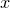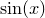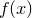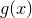# 8.5 Plotting functions in exotic styles

The use of plot styles which take more than two columns of input data to plot functions requires more than one function to be supplied. When functions are plotted with syntax such as

plot sin(x) with lines


two columns of data are generated: the first contains values of– plotted against the horizontal axis – and the second contains values of– plotted against the vertical axis. Syntax such as

plot f(x):g(x) with yerrorbars


generates three columns of data. As before, the first contains values of. The second and third contain samples from the colon-separated functionsand. Specifically, in this example,provides the uncertainty in the value of. The using modifier may also be used in combination with such syntax, as in

plot f(x):g(x) using 2:3


though this example is not sensible.would be plotted on the y-axis, againston the x-axis. However, this is unlikely to be sensible because the range of values ofsubstituting into these expressions would correspond to the range of the plot’s horizontal axis. The result might be particularly unexpected if the above were attempted with an autoscaling horizontal axis – Pyxplot would find itself autoscaling the x-axis range to the spread of values of, but find that this itself changed depending on the range of the x-axis. In this case, the user should have used the parametric plot option described in the next section.